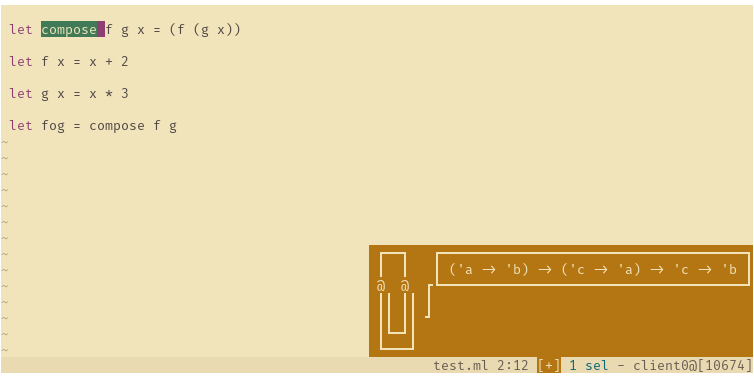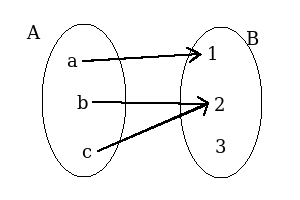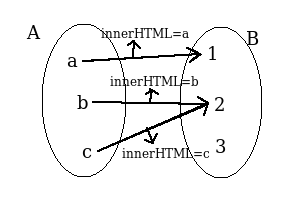# A look into composing, Composability, Immutability

August 2019 · 12 minute read

An ordinary work day,
I’m adding a feature to our JavaScript driven tables so it can pull/push data while navigating between the pages(I belive in common tongue they call this "pagination"). I wrote two functions named `retrieveItems` and `drawItems`. As I’m building the `retrieveItemsAndDraw` function, this is what I find myself writing:

``const retrieveAndDrawItems = R.compose(drawItems, retrieveItems)``

At that time one of the guys in my team creep up behind me and ask what I’m up to, and what that line does. "Simple!" I answer, "It’s just `myCompose = fs ⇒ x0 ⇒ fs.reverse().reduce((x, f) ⇒ f(x), x0)`~".

But that answer isn’t correct. And `compose` (or function composition) as simple as it may be is a fundamental concept and need to be understood and explained properly. This text has been written for the sake of `myCompose`; to understand it and to explain it in detail.

## Composing functions

Do you remember function composition from mathematics? It’s when you join multiple functions together to create a new one. Joining, or compose or composability is the bread and butter of functional programming in achieving modularity.

f,g,fog
```f(x) = x + 2
g(x) = x * 3
(fog)(x) = f(g(x)) = (3 * x) + 2```
f,g,fog JavaScript’te
``````f = x => x + 2
g = x => x * 3
fog = x => f(g(x))``````

### Building the compose function

My next step is to write a generic compose function; a function that takes two functions and joins them; but first I would like to be able to describe the inputs and outputs of those functions so I can argue about how they can be joined together.

In functional languages, function signatures resemble that of Haskell:

1. `string → int` : A function. Takes `string` and returns `int`

2. `f :: string → int`: The function named `f` takes `string` and returns `int`

3. `int → int → int`: A function that takes 2 integers and returns one integer

4. `indexOf: [string] → int`: a function named `indexOf` that takes `string` list and returns an `int`

5. `find :: ['a] → Maybe 'a`: a function that takes a list of a generic type(`a`), and returns a `Maybe` container with a value of that same type (`a`) in it

Being able to read function signatures will help me argue about which functions I can compose together and how. Lets return to a a simple composition example to see how:

f,g,fog in JavaScript
``````f = x => x + 2
g = x => x * 3
fog = x => f(g(x))``````

If I know what type inputs and outputs `f` and `g` have, then I can know what their composition will input and output:

```f :: 'a -> 'b
g :: 'b -> 'c
fog :: 'a -> 'c```

Or to give more concrete examples:

``````//myParseInt :: string -> int
myParseInt = parseInt (1)

//indexOf :: 'a -> ['a] -> int
indexOf = x => xs => xs.indexOf(x)

//increment :: int -> int
increment = x => x + 1

//uppercase :: string -> string
uppercase = x => x.toUpperCase()

// cities :: [string]
cities = ["ANKARA", "ISTANBUL", "KAYSERI"]

// indexOfCity :: string -> int
indexOfCity = x => indexOf(uppercase(x)) (2)``````
 1 `parseInt` would return `NaN` for things that it cannot parse into numbers, but lets ignore that for the moment 2 the function `indexOfCity` is `indexOf` and `uppercase` put together. As in the case of `(fog)(x)` but with `indexOf` in the place of `f` , `uppercase` in the place of `g`.

Similarly, we can see `uppercase(myParseInt("4"))` is faulty but `increment(myParseInt("4"))` is not.

As I said, splitting a problem into functions and creating solutions that are composed of those functions is the basic functional approach to programming. Which is why in `Haskell` to compose two functions into one is as easy as typing `.`:

``indexOfCity = (indexOf . uppercase)``

In JavaScript however we have to roll our own:

f,g,fog in JavaScript
``````//compose :: (('b -> 'c), ('a -> 'b)) -> 'a -> 'c  (1)
compose = (f, g) => x => f(g(x))

f = x + 2
g = x * 3
fog = compose(f, g)``````
 1 If that is looking complicated I suggest you pause and wait till it clicks until you proceed further

In fact, if I did the same in OCaml and queried the type of `compose` I would get:In OCaml to call a function named `f` with the arguments `x` and `y`, instead of the familiar `f(x, y)` we say `f x y`. Operators like `+` and `-` come between its two arguments, as in `x + 2`. We can create infix methods (like `a + b`) as well as prefix ones (like `(+) a b`) if we desired: ``````let (><) f g x = f (g x) let f x = x + 2 let g x = x * 3 let fog = f >< g`````` But in OCaml the pipe operator (`|>`) is preferred to `compose`: ``let fog x = x |> g |> f`` There is also a proposition to have the `|>` operator added to ECMA
 In Kakoune, the command `:lsp-hover` can show me the signature of whatever my cursor is on

### A general purpose compose method

Sometimes I feel like composing more than two functions together. In JavaScript this is also trivial to accomplish:

an innocent piece of code that tries to compose functions
``````compose = fs => x0 => (fs (1)
.reverse() (2)
.reduce((x, f) => f(x), x0)
)``````
 1 I could have got variable number of arguments instead of an array by declaring the function as `compose = (…​fs) ⇒ …​` 2 Because `compose([f, g])(x)` means `f(g(x))` , not `g(f(x))` ; I reverse the list. `reduce` would iterate over the list from the beginning to the end.

Did anyone else have their spider senses tingling after reading the `compose` function above?

 The `compose` above DOES NOT WORK

But why does it not work?

``````const f = x => x + 2
const g = x => x * 3
const h = x => x * x

const hfg = compose([h, f, g])

hfg(5) // 289
hfg(5) // 81
hfg(5) // 289
hfg(5) // 81
//And it continues forever in the same pattern``````

Have you realized that `hfg` does `h(g(f(x)))` first, and `f(g(h(x)))` after?

## Immutability

``````const f = x => x + 2
const g = x => x * 3
const h = x => x * x

const funs = [h, f, g]
const hfg = compose(funs)

hfg(5) //result 289
console.log(funs) // [ [Function: g], [Function: f], [Function: h] ]
hfg(5) //result 81
console.log(funs) // [ [Function: h], [Function: f], [Function: g] ]
hfg(5) //result 289``````

Is it obvious yet?

The `reverse` method of `Array` modifies the array it is called on.
So it turns out `reverse` is mutative.

## Mutative Function

Mutative function simply does what its name suggests; it may mutate the program state

To get into what state is I believe it’s best to know what a pure function is.

 In Ruby the mutative functions have a `!` at the end of their names. If a function is named `reverse!` instead of `reverse` you very well know that it modifies something somewhere.

### Pure Function

In mathematics (if you remember) a function is just a relation between two sets.eg.a function from A to B

Lets call this function `fab` and write it in JavaScript:

``````const A2B = { a: 1, b: 2, c: 2 }
fab = x => A2B[x]``````

Like how `fab` maps A to B, `f(x) = 2x + 3` is a function that maps natural numbers to natural numbers.eg:`f(x) = 2x + 3` function mapping Z to Z

Functions like `fab` and `f(x) = 2x + 3` that only map one set to the other are called pure . The definition pure function may make more sense knowing what a side effect is:

### Side Effect

Lets say our `fab` method does not only map A to B, it does more; it renders a text in a webpage:

``````fabAndEdit = x => {
document.querySelector("#text").innerHTML = x
return A2B[x]
}``````eg:fabAndEdit

Like the setting of `innerHTML`, any effect that is not a mapping of an input to an output is called a side effect.

You can also think of a side effect as anything that changes the program state.

State is the state of a program or a computer. For example when you press a button on your keyboard, you change the state of your computer from a computer to a computer-that-has-a-button-pressed. If you modify a textbox the state of the program is changed, something writes in that textbox that didn’t write before; if a different piece of code were to read the value of that textbox after it has been modified it will find a different value than it would before.

• Functions that don’t modify the state are called pure functions.

• If a function is modifying the state that modification is called a side effect.

• Pure functions don’t have side effects. Functions that have side effects are not pure.

• Pure functions always give the same result for the same input no matter when they are called.

• Mutative functions have side effects. Mutative functions are not pure.

### The Mental Overhead of Mutative Functions

The number of objects an average human can hold in working memory is 7±2

— George A. Miller
The Magical Number Seven

Our mental capacity is not limitless; and if you are like me you’re listening to a podcast with one ear, while the other ear is focused on the sounds coming from the meeting room to check if anyone is saying anything about you; while you’re writing code.

In addition for every function in your code, instead of having to ponder if and how they’re modifying the program state, how they’re modifying the global variables, if they overwrote your function arguments; if you wrote them pure, if you limited their side effects (if any) it would make your code more maintainable (then again, there is no program that doesn’t have side effects; what’s the point of a program if it doesn’t take an input and it doesn’t produce an output. We could even get philosophical and argue that if we can’t see the output of a program, it does not exist).

Side effects can be purified by making them lazy.

Let me explain what that means…​

If a function doesn’t produce its output immediately, if the result is delayed until the moment it is needed that function is called lazy.

Haskell, for example, is an inherently lazy language. In OCaml we can have lazy values by declaring them `lazy`. In JavaScript (as usual) we have to hack our own:

``````counter = 0

incrCounter_unsafe = () => {counter ++}
incrCounter = () => () => {counter ++}

range = n => { let l = []
for (let i = 0; i < n; i ++) { l.push(i) }
return l }

range(3) (1)
console.log(counter) (2)
range(3).forEach(incrCounter_unsafe)
console.log(counter) (3)

counter = 0
lazyFuns = range(4).map(incrCounter)
console.log(counter) (4)
lazyFuns.forEach(f => f())
console.log(counter) (5)``````
 1 `[0, 1, 2]` 2 prints `0` 3 prints `3` 4 prints `0` 5 prints `4`

`incrCounter_unsafe` is impure, because it modifies the global variable `counter`. `incrCounter_unsafe` upon being called does what it must: it immediately changes the value of `counter`.

On the other hand `incrCounter` function doesn’t do a thing when it is called, it just says "hey man…​ I really can’t be bothered right now. Here just take this and you just call it yourself, won’t you?" returning a function. No change happens in the program state until the returned function is called; which is why `incrCounter` is safe and lazy.

An all-purpose solution for things lazy would be:

``````class LazyF {
constructor(f)   { this.$f = f } // map :: LazyF ('a -> 'b) -> ('b -> 'c) -> LazyF ('a -> 'c) map(f) { return new LazyF(x => f(this.$f(x))) }
// chain :: LazyF ('a -> 'b) -> LazyF ('b -> 'c) -> LazyF ('a -> 'c)
chain(o) 	     { return new LazyF(x => o.performUnsafe(this.$f(x))) } // performUnsafe :: LazyF ('a -> 'b) -> 'a -> 'b performUnsafe(x) { return this.$f(x) }
}

foo = x => {console.log("x: " + x)
return x + 1}
foo(5) (1)
new LazyF(foo) (2)
new LazyF(foo).performUnsafe(5) (3)
new LazyF(foo).map(foo).performUnsafe(5) (4)

counter = 0
//incrCounter :: () -> LazyF (() -> ())
incrCounter = () => new LazyF(() => {counter ++})
lazy = (incrCounter()
.chain(incrCounter())
.chain(incrCounter()))
console.log(counter) (5)
lazy.performUnsafe()
console.log(counter) (6)``````
 1 prints `x: 5` and returns `6` 2 `LazyF {'\$f': [Function: foo]}` 3 prints `x: 5` and returns `6` 4 prints `x: 5` and `x: 6` , returns `7` 5 prints `0` 6 prints `3`
 Haskell can be a purely functional programming language by wrapping every side effect in containers called `IO`
 A similar solution to `LazyF` can be found in a JavaScript library named `crocks` , under a different name: `Arrow`

### Mutability, Immutability

A term that goes hand-in-hand with "mutative". Compare the behaviour of the Array in JavaScript to that of ImmutableArray

While `push` method of Array modifies values, the `push` method in `ImmutableArray` doesn’t. Data types like ImmutableArray that protect their states are called immutable. Immutable values also encourage purification of the functions they are used in

 In functional languages you don’t have to struggle to achieve immutable values; they are supported natively. For example in OCaml the concept of variable doesn’t exist. To think about it the name "variable" in mathematics actually contradicts with what it actually is; you can’t declare `g = 9.8` in the beginning of your formula and half way through change `g` to something else; if a variable doesn’t vary, then it isn’t a variable is it? In OCaml once you say `let a = 5` , `a` is always a label for the value `5`. If you want modifiable values you could `let counter = ref 5` and then `counter ← !counter + 1`. For records: ocaml mutable records ``````type sex = Male | Female type person = {name: string; age: int; sex: sex; } let a_person = {name="Bruce"; age=60; gender=Male} a_person.sex <- Female (* ERROR *) let a_new_person = {a_person with name="Caitlynn"; gender=Female} (* OK *) type changable_person = {mutable name: string; age: int; mutable sex: sex; } let a_person2 = {name="Bruce"; age=60; gender=Male} a_person2.name <- "Caitlynn" a_new_person <- Female`````` If you too want to write code with immutable values but having your JavaScript focused team switch to OCaml is out of the picture, you may want to try immutableJS: a library that provides immutable implementations of many collection data types If your answer is "thanks but no thanks" then I can only wish you luck with your endless `clone()`ing and `slice(0)`ing…​

## Uhm, Conclusion?

In conclusion we have a working `compose` method:

compose
``````reverseList = l => l.slice().reverse()

compose = (...fs) => x0 => reverseList(fs).reduce((x, f) => f(x), x0)``````

Of course the kid that asked me what `R.compose` does has long gone…​ He didn’t stick around to listet to my storytelling. That’s OK though, I’ll mansplain to him tomorrow continuing from where we left off…​